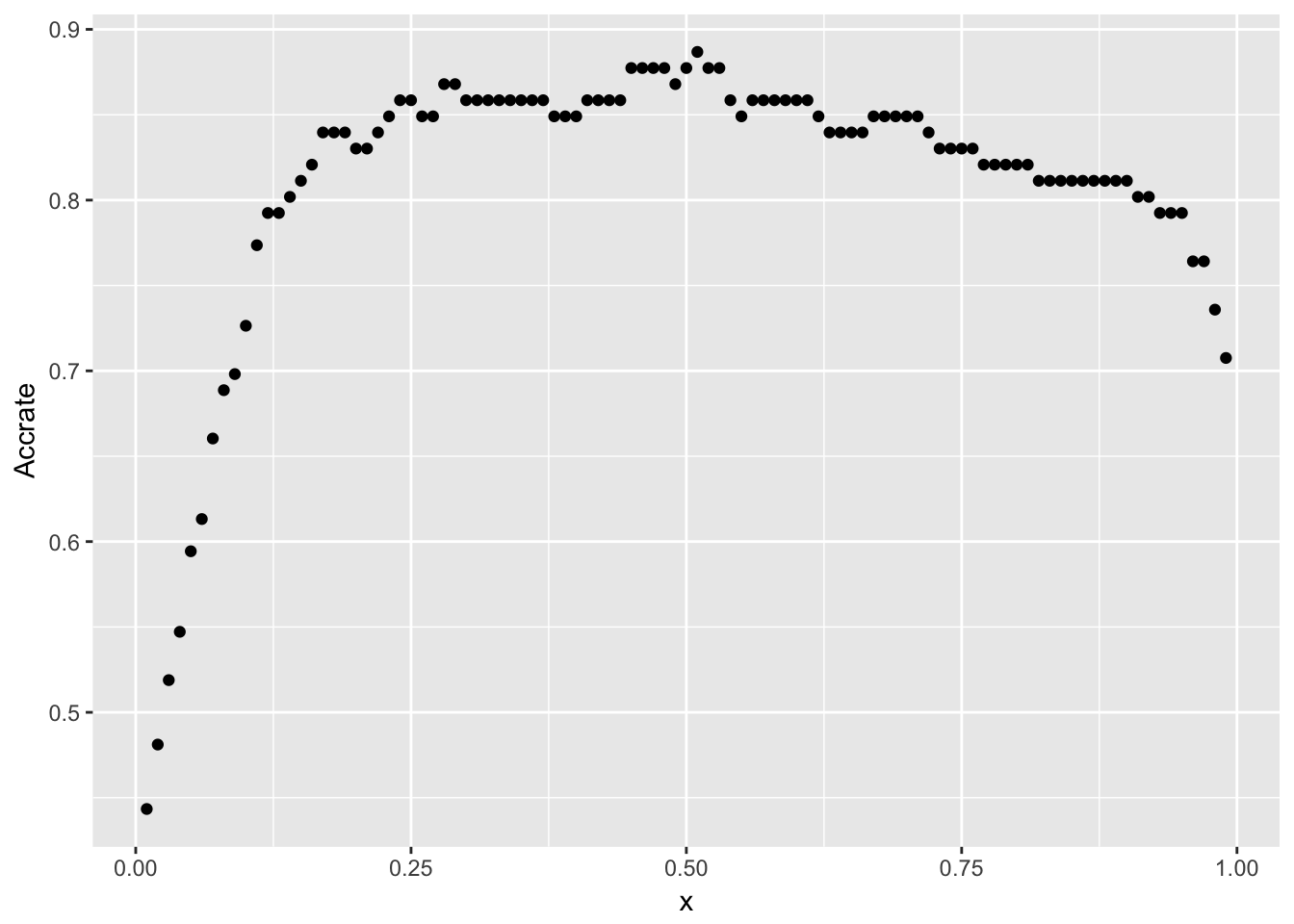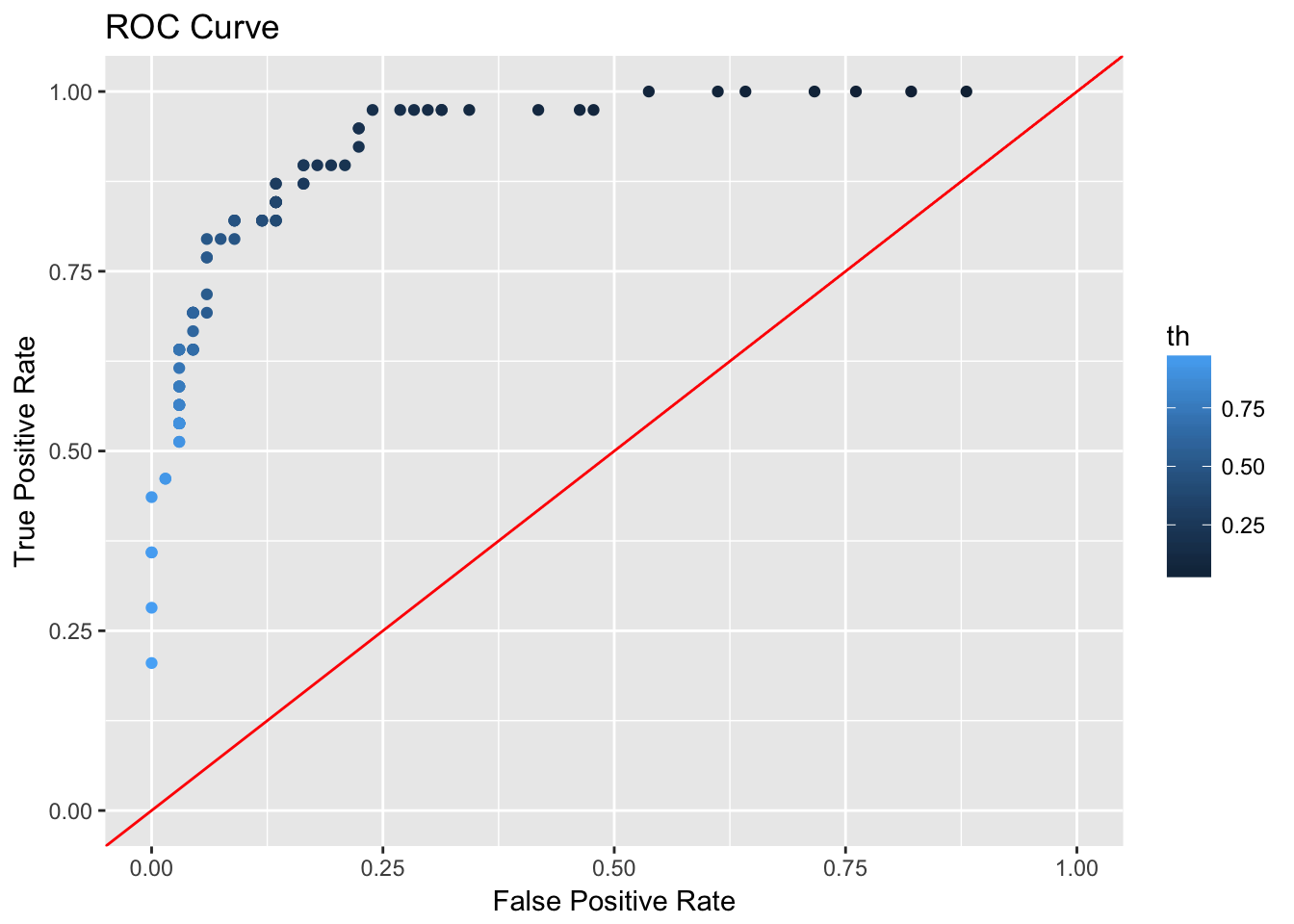# An Example of a Logistic Model

``library(openintro)``
``## Please visit openintro.org for free statistics materials``
``````##
## Attaching package: 'openintro'``````
``````## The following objects are masked from 'package:datasets':
##
##     cars, trees``````
``library(tidyverse)``
``````## Loading tidyverse: ggplot2
``## Conflicts with tidy packages ----------------------------------------------``
``````## filter(): dplyr, stats
## lag():    dplyr, stats``````
``library(broom)``

## Create a binary variable that marks counties in the state of Washington. Also keep only counties from Alabama or Washington to make the problem easier.

``````countyComplete %>%
filter(state %in% c("Washington","Alabama")) %>%
mutate(WA = state =="Washington") -> ccm

# Make sure it worked
table(ccm\$WA)``````
``````##
## FALSE  TRUE
##    67    39``````

## Build a Model

The model should identify counties in Washington based on characteristics in the countyComplete (ccm) dataframe.

``````WAMod = glm(WA ~ area + poverty + median_household_income,data=ccm,family=binomial)
summary(WAMod)``````
``````##
## Call:
## glm(formula = WA ~ area + poverty + median_household_income,
##     family = binomial, data = ccm)
##
## Deviance Residuals:
##     Min       1Q   Median       3Q      Max
## -2.4225  -0.4632  -0.2236   0.2105   2.2696
##
## Coefficients:
##                           Estimate Std. Error z value Pr(>|z|)
## (Intercept)             -4.670e+00  4.718e+00  -0.990    0.322
## area                     3.428e-03  8.737e-04   3.923 8.74e-05 ***
## poverty                 -1.766e-01  1.427e-01  -1.237    0.216
## median_household_income  8.484e-05  6.864e-05   1.236    0.216
## ---
## Signif. codes:  0 '***' 0.001 '**' 0.01 '*' 0.05 '.' 0.1 ' ' 1
##
## (Dispersion parameter for binomial family taken to be 1)
##
##     Null deviance: 139.462  on 105  degrees of freedom
## Residual deviance:  65.368  on 102  degrees of freedom
## AIC: 73.368
##
## Number of Fisher Scoring iterations: 6``````

## Check Performance

``````ProbWA = predict(WAMod,type="response")
PredWA = ProbWA > .5

# Create the confusion matrix
table(PredWA,ccm\$WA)``````
``````##
## PredWA  FALSE TRUE
##   FALSE    62    8
##   TRUE      5   31``````
``````# Compute the overall accuracy rate
AccRate = mean(PredWA == ccm\$WA)
AccRate``````
``##  0.8773585``

Of the 39 counties in Washington, 31 were classified correctly and 8 were incorrectly identified as Alabama counties. In the case of Alabama, 62 counties were identified correctly and 8 were classified as Washington counties.

The overall accuracy was about 88%.

## Vary the Threshold

``````PredWA = ProbWA > .3

# Create the confusion matrix
table(PredWA,ccm\$WA)``````
``````##
## PredWA  FALSE TRUE
##   FALSE    58    6
##   TRUE      9   33``````
``````# Compute the overall accuracy rate
AccRate = mean(PredWA == ccm\$WA)
AccRate``````
``##  0.8584906``

## Create and Use a Function fAccrate

``````fAccrate = function(th){
PredWA = ProbWA > th
return(mean(PredWA == ccm\$WA))
}
x = seq(from = .01,to=.99,by = .01)
Accrate = sapply(x,fAccrate)
Accrate``````
``````##   0.4433962 0.4811321 0.5188679 0.5471698 0.5943396 0.6132075 0.6603774
##   0.6886792 0.6981132 0.7264151 0.7735849 0.7924528 0.7924528 0.8018868
##  0.8113208 0.8207547 0.8396226 0.8396226 0.8396226 0.8301887 0.8301887
##  0.8396226 0.8490566 0.8584906 0.8584906 0.8490566 0.8490566 0.8679245
##  0.8679245 0.8584906 0.8584906 0.8584906 0.8584906 0.8584906 0.8584906
##  0.8584906 0.8584906 0.8490566 0.8490566 0.8490566 0.8584906 0.8584906
##  0.8584906 0.8584906 0.8773585 0.8773585 0.8773585 0.8773585 0.8679245
##  0.8773585 0.8867925 0.8773585 0.8773585 0.8584906 0.8490566 0.8584906
##  0.8584906 0.8584906 0.8584906 0.8584906 0.8584906 0.8490566 0.8396226
##  0.8396226 0.8396226 0.8396226 0.8490566 0.8490566 0.8490566 0.8490566
##  0.8490566 0.8396226 0.8301887 0.8301887 0.8301887 0.8301887 0.8207547
##  0.8207547 0.8207547 0.8207547 0.8207547 0.8113208 0.8113208 0.8113208
##  0.8113208 0.8113208 0.8113208 0.8113208 0.8113208 0.8113208 0.8018868
##  0.8018868 0.7924528 0.7924528 0.7924528 0.7641509 0.7641509 0.7358491
##  0.7075472``````
``````result = data.frame(x,Accrate)
result %>% ggplot(aes(x,Accrate)) + geom_point()````````````winner = which(Accrate == max(Accrate))
winner``````
``##  51``
``x[winner]``
``##  0.51``

## Is Overall Accuracy Everything?

We may have asymmetric concerns about false positives and false negatives.

If the probability that a plan is edible is .5, would you call the plan edible?

How do you compute the false positive and false negative rates?

Use the confusion matrix.

``````th = .5
PredWA = ProbWA > th
cmat = table(PredWA,ccm\$WA)
cmat``````
``````##
## PredWA  FALSE TRUE
##   FALSE    62    8
##   TRUE      5   31``````
``cmat[1,1]``
``##  62``

## Be Systematic

We could type different threshold values into this code snippet and observe effects on the FPR and FNR. Or we could build functions and use them to show how the values of FPR and FNR respond to th.

Hint: That’s an exercise. Use my code for fAccrate as a template.

Note: The code here is based on two assumptions.

• The confusion matrix has predictions in rows and actuals in columns.

• The values are either 0,1 or FALSE, TRUE so that the rows and columns are sorted correctly.

``````f_tpr = function(th){
PredWA = ProbWA > th
cmat = table(PredWA,ccm\$WA)
return(cmat[2,2]/(cmat[1,2] + cmat[2,2]))
}

f_fpr = function(th){
PredWA = ProbWA > th
cmat = table(PredWA,ccm\$WA)
return(cmat[2,1]/(cmat[2,1] + cmat[1,1]))
}

# Create the three vectors we need

th = seq(from=.01,to=.99,by=.01)
fpr = sapply(th, f_fpr)
tpr = sapply(th,f_tpr)

# Put the vectors into a dataframe so we can use ggplot2.

df = data.frame(th,tpr,fpr)

# The most important curve is the Receiver Operating Characteristic curve. It describes the tradeoff between sensitivity and specificity.

df %>% ggplot(aes(fpr,tpr)) +
geom_point(aes(color=th)) +
ggtitle("ROC Curve") +
xlab("False Positive Rate") +
ylab("True Positive Rate") +
geom_abline(slope=1,intercept=0,color="red") +
scale_x_continuous(limits=c(0,1)) +
scale_y_continuous(limits=c(0,1))````````````df %>% ggplot(aes(x=th,y=fpr)) +
geom_point() +
ggtitle("False Positive Rate ~ Threshold") +
xlab("Threshold") +
ylab("False Positive Rate")``````